Mathematical and Physical Journal
for High Schools
Issued by the MATFUND Foundation
 Already signed up? New to KöMaL?

# KöMaL Problems in Physics, March 2012

Show/hide problems of signs:## Problems with sign 'M'

Deadline expired on April 10, 2012.

M. 322. Measure how long it takes to a single pulse generated at one end of a horizontal Slinky, to travel back to the place where it was initiated, if the Slinky is stretched differently. Examine how this time depends on the distance between the turns.

(6 pont)

statistics## Problems with sign 'P'

Deadline expired on April 10, 2012.

P. 4423. Can the energy of a charged condenser be increased without giving more charge to it?

(3 pont)

solution (in Hungarian), statistics

P. 4424. A 20-litre plastic can contains petrol. The can is floating on the surface of the water of a lake, such that one-third of the volume of the can is out of the water. The mass of the empty can is 0,78 kg. The density of petrol is 720, the density of plastic is 1300. Approximately how many litres of petrol are there in the can?

(4 pont)

solution (in Hungarian), statistics

P. 4425. A small object is mounted to the perimeter of a hoop of radius r. The mass of the object and the hoop is the same. The hoop is placed into a fixed semi-cylinder shaped rough trough of radius R, such that the small mass is at the top. Find the least r/R ratio such that the equilibrium state of the hoop is stable.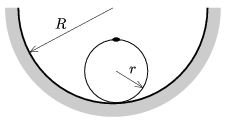(6 pont)

solution (in Hungarian), statistics

P. 4426. On a smooth horizontal tabletop there is a spring, one of its end is braced, and at the other end there is a ball of mass M=1.15 kg at rest. Another ball of mass m=1.00 kg hangs on a vertical thread of length=0.30 m and touches the previoussly mentioned one. The ball on the thread is displaced to the horizontal and released without any initial speed. The velocity of the ball at which it reaches the horizontal is parallel to the symmetry axis of the spring. The collision of the two balls is totally elastic. a) What should the spring constant of the spring be, in order that the second collision of the balls occurs at the same position as the first one did? b) How much time elapses between the two collisions? c) After the first collision what is the maximum distance between the two balls? (Friction and the mass of the spring are negligible.)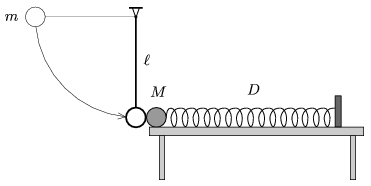(5 pont)

solution (in Hungarian), statistics

P. 4427. An iron rod of length L is hung at a common point with threads of lengthwhich are attached to the two ends of the rod. The rod is displaced a bit in the plane of the threads. What is the length of the threads if the period of the swinging of the rod is the least, and what is this period?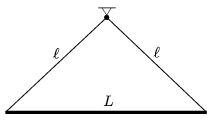(5 pont)

solution (in Hungarian), statistics

P. 4428. In two containers of equal volume there are two samples of gas: Helium and Hydrogen. The samples have equal internal energy and equal number of particles. a) What is the ratio of the pressure of the gases? b) What is the ratio of the average speed of the particles?

(4 pont)

solution (in Hungarian), statistics

P. 4429. It is not suggested to turn off the projectors used nowadays. After the lamp was switched off the cooling fan is working for some minutes and only then the projector is allowed to unplug. Edith states that this is unnecessary, If the lamp is not on, it cannot be warmer, and the lamp endures at working temperature. So if we do not want to use the projector, it can be unplugged.'' Is Edith right?

(4 pont)

solution (in Hungarian), statistics

P. 4430. In the circuit shown, R=1 k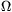, C=10 mF. What is the terminal voltage of the battery if the condenser stores 4,5 J energy after it was charged?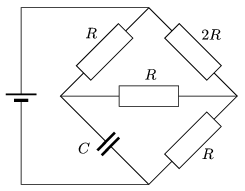(4 pont)

solution (in Hungarian), statistics

P. 4431. One side of an old phonograph record was thoroughly wiped, thus this side was charged evenly to a charge of Q=1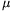C. The phonograph rotated the record of diameter d=300 mm, the number of revolution of the turntable was f=78/minutes. What is the magnetic induction at the centre of the record? Compare the orders of this magnetic induction and the magnetic induction of the Earth.

(5 pont)

solution (in Hungarian), statistics

P. 4432. Two lenses are placed such that their principle axes are common, and they are at a distance of 5 cm. One of them is a converging lens of optical power of 10 dioptres and the other is a diverging lens of optical power of -5 dioptres. A small filament lamp is placed 20 cm from the converging lense, not on the side at which there is the diverging lens. Where should the screen be placed in order to gain the sharp image of the filament on it? Where will the image be if the filament lamp is placed closer to the converging lens by one-sixth of the initial 20 cm distance?

(4 pont)

solution (in Hungarian), statistics

P. 4433. If a certain heavy metal is illuminated by UV light of wavelength of 185 nm, electrons are ejected from it at a speed of 1.00.106 m/s. a) Which is this metal, and who discovered it? b) What is the speed of the electrons if UV light of wavelength 254 nm is used to illuminate it?

(4 pont)

solution (in Hungarian), statistics